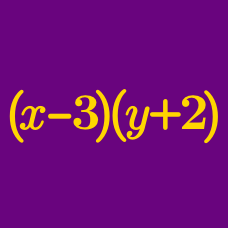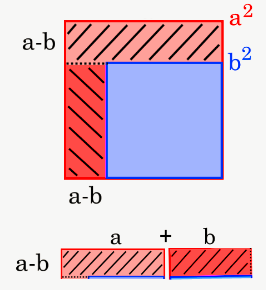Algebra

Polynomial Arithmetic: Level 2 Challenges$\large \frac{ 11^4 - 9 ^ 4 } { 11 ^2 + 9^ 2 } = \ ?$

$\large \left( \sqrt{{\color{#D61F06}60}} + {\color{#3D99F6}3}\sqrt{{\color{#3D99F6}7}}\right)^2\left( \sqrt{{\color{#D61F06}60}} - {\color{#3D99F6}3}\sqrt{{\color{#3D99F6}7}}\right)^2 = \ ?$

$\large x+\frac{1}{x} = 5, \quad\quad\quad x^2 + \frac{1}{x^2} = \ ?$

$\large 10^x=\Big(10^{624}+25\Big)^2-\Big(10^{624}-25\Big)^2$

What is the value of $x$ that satisfies the equation above?

Which of the following equals $\dfrac { { a }^{ 2 }-{ b }^{ 2 } }{ a-b }$ for $a\neq b$?

×